##### Difference Between DIV() and MOD() Function in PostgreSQL

PostgreSQL provides various built-in mathematical and trigonometric functions to deal with numeric data. For instance, the ROUND() function rounds a number; the RANDOM() function generates the random numbers, the abs() function retrieves the absolute value, etc. The DIV() and MOD() are also mathematical functions that perform the division on the given values.

This post uses practical examples to present a comparative analysis of the Postgres MOD() and DIV() functions.

Difference Between DIV() and MOD() Function in Postgres

The DIV() and MOD() are built-in math functions that accept the numeric values and perform division on them. However, the DIV() function retrieves a quotient while the MOD() function retrieves the remainder.

Let’s put these functions into practice for a profound understanding.

Example 1: How Does the DIV() Function Work in Postgres?

In the following example, we will pass two numeric values to the DIV() function:

`SELECT DIV(5000, 4);`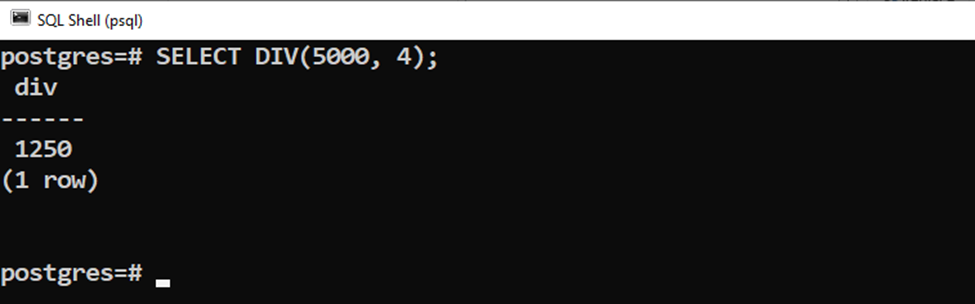The output shows that the DIV() function retrieves the quotient integer.

Example 2: How Does the MOD() Function Work in Postgres?

In the following example, we pass the same numeric values to the MOD() function:

`SELECT MOD(5000, 4);`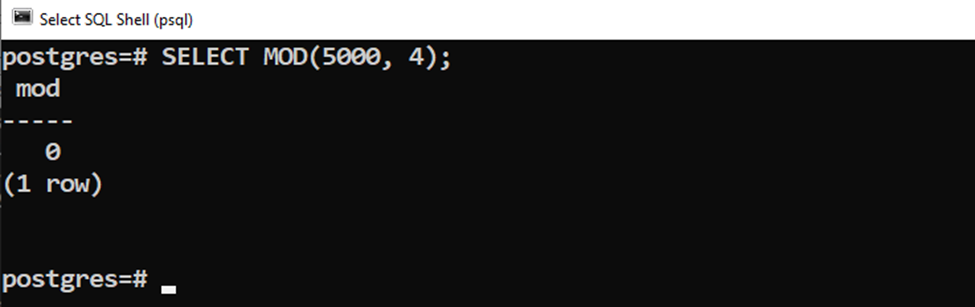The output shows that the MOD() function retrieves the remainder instead of a quotient integer.

Example 3: How Does the DIV() Function Work With Negative Values in Postgres?

Let’s pass a negative value to the DIV() function and see how the DIV() function treats the negative values:

`SELECT DIV(-5000, 4);`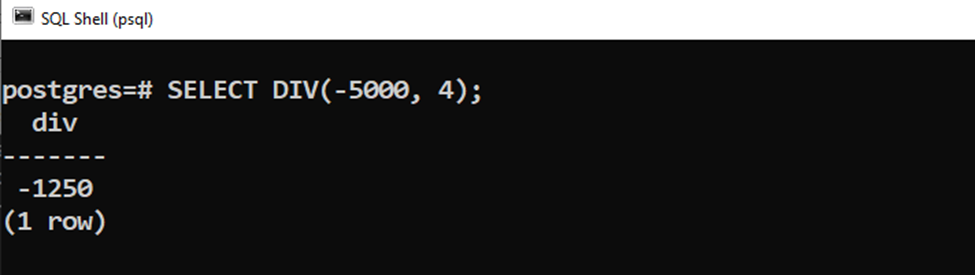The output clarifies that this time the DIV() function returns a negative quotient.

Example 4: How Does the MOD() Function Work With Negative Values in Postgres?

Let’s pass a negative value to the MOD() function and see how it works:

`SELECT MOD(-5000, 3);`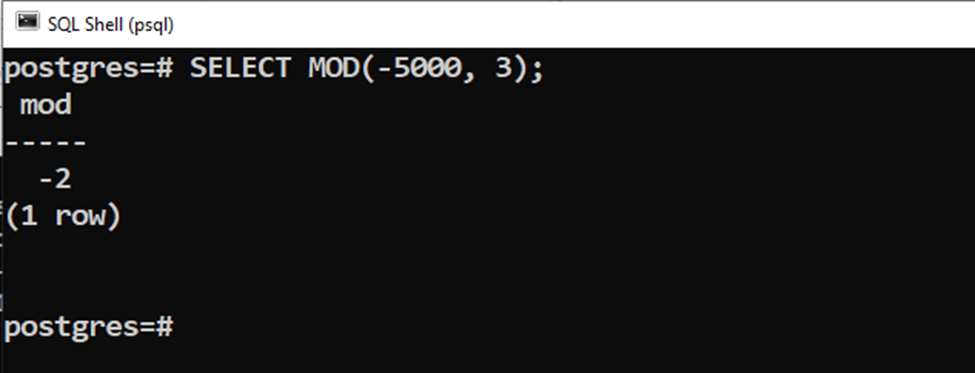The MOD() function retrieves the remainder with the negation sign.

Example 5: How Do the DIV() and MOD() Functions Work on Table’s Data?

We have a sample table named “example_data” that contains the following data: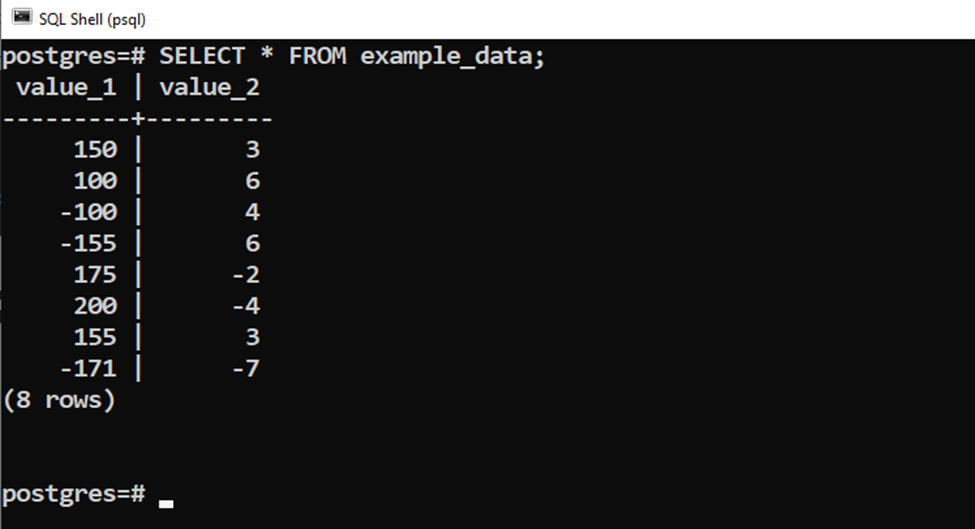Let’s learn how to use the DIV(), and MOD() functions on the table’s data:

```SELECT value_1, value_2,
DIV(value_1, value_2) AS div_result,
MOD(value_1, value_2) AS mod_result
FROM example_data;```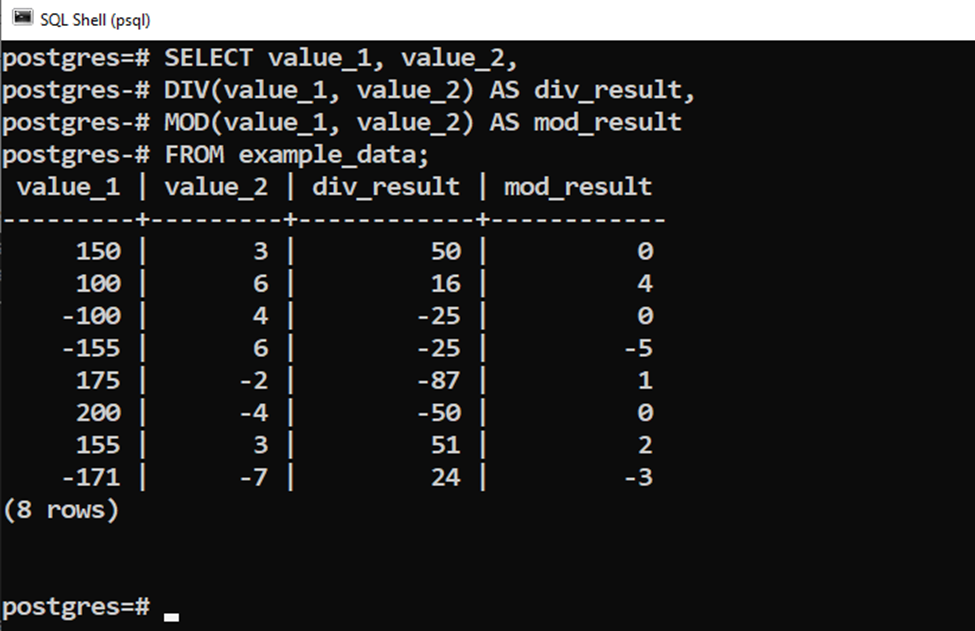The output shows that when both the numerator and denominator are negative, the DIV() function retrieves a positive value, while the MOD() function retrieves a negative value.

This is how the DIV() and MOD() functions work on Postgres tables.

Conclusion

The DIV() and MOD() are built-in math functions that accept the numeric values and perform division on them. However, the DIV() function retrieves a quotient while the MOD() function retrieves the remainder. When both the numerator and denominator are negative, the DIV() function retrieves a positive value, while the MOD() function retrieves a negative value. This Post explained the difference between DIV() and MOD() functions using suitable examples.